# Denoising

Important: Brevity disclaimer.

## Introduction

Basically, denoising (also sometimes called smoothing) is about decreasing the noise present on the mesh. Quite often the noise is present due to inaccuracies of mesh construction by scanning processes, such as CT scanning.

Task of mesh denoising is quite well studied area of research - there are a lot of algorithms ranging from simple Laplacian denoising (AKA just averaging neighbor points) to more sophisticated schemes, involving simultaneous operations on all vertices using iterative linear systems solver based on sparse matrices.

## Volume compensation

One very important (and typically, undesirable) effect of most of the denoising algorithms is so called “shrinking”, which means change of the mesh volume (most often - its decrease) during denoising process.

It’s easy to understand the nature of this effect. Suppose that we perform denoising as calculating for each point the average position of all its neighbor points, and move our point towards this averaged one.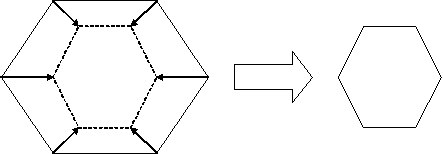Arrows on the picture show directions and end points where original points are moved to, thus producing the shape on the right. For actual meshes, this situation does not happen for all 100% of vertices, as sometimes averaged point position lies outside of the shape, not inside, but nonetheless this is true for the most vertices, thus producing shrinkage in the result.

This means that “shrinking” is in some sense inherent part of denoising process, so it has to be compensated using some additional processing, and this processing may partially “undo” denoising effect also - so, resulting mesh will be less smooth than it could be without volume compensation when using the same denoising parameters. Typically, however, this is also the desired property of volume compensation, as it tends to cancel “global” changes rather than “local” ones, thus allowing to eliminate the noise, while leaving “global” mesh features unchanged.

There were several attempts to solve “shrinking” problem in the past via using some kind of scaling, or two steps denoising (such as Taubin denoising), but all these algorithms were too primitive, too slow or too difficult to use for the end user.

Key points of the algorithm developed for Materialise are:

• Performing very accurate volume compensation - down to numerical accuracy of calculations, typical difference in volumes between original and result mesh is less than 0.01% independent on denoising strength.
• Almost zero overheads, both for speed and memory.
• No additional input from the user required other than turning the option of volume compensation on.

Some examples of algorithm results follow.

## Sphere denoising

Though this example is useless from practical point of view, sphere denoising is often used to demonstrate some basic properties of denoising algorithm: while no normal denoising algorithm can make the ideal sphere smoother than it is, it is important that algorithm at least does not make things significantly worse.

### Original sphere### Sphere after denoising without volume compensation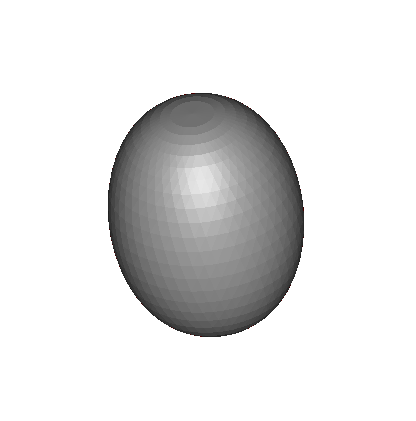### Sphere after denoising with volume compensation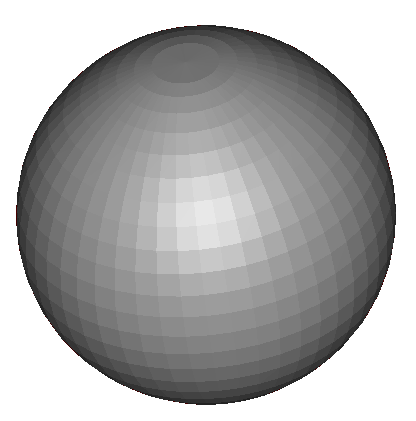In last two cases, sphere was processed using exactly the same denoising algorithm parameters, except that volume preservation was not performed in the first call.

Volume difference without volume compensation here is about 81%. With volume compensation turned on, the difference is about 0.004%.

## BallJoint model denoising

Model is courtesy of Cyberware, Inc.

### Original model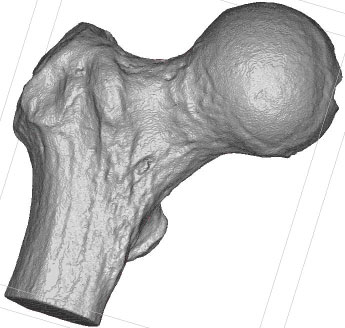### Denoising result without volume compensation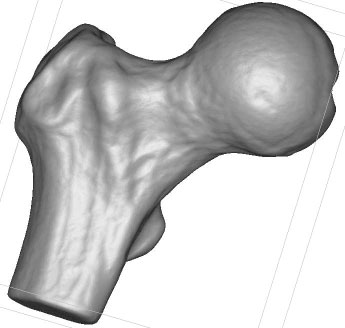### Denoising result with volume compensation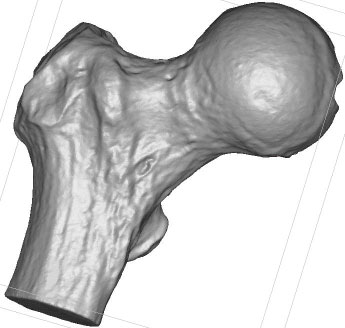### Original model (close up)### Denoising result without volume compensation (close up)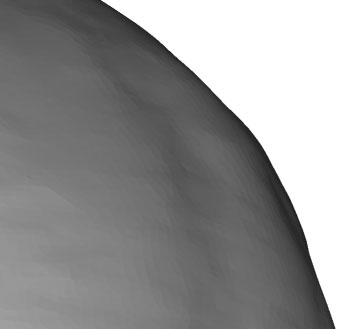### Denoising result with volume compensation (close up)Volume difference without volume compensation here is about 1%. With volume compensation turned on, volumes match exactly down to the accuracy of used model representation (so, the difference, if exists, is less than about 0.0001%).

Please notice the differencies in close ups pictures: while no volume compensation provides visually more “smooth” mesh surface, it can be seen that some surface details were smoothed out, while with volume compensation turned on, these features are more visible, yet the noise is not present.